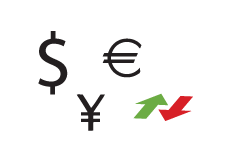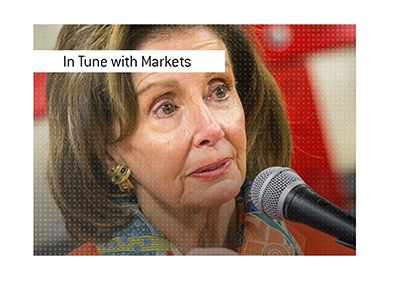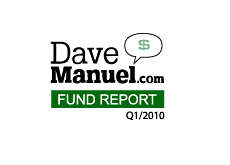Understanding how forex trades work can be a little tricky, even for the person who has had experience trading stocks.

There are pips, lots, base currencies and counter currencies. What does it all mean?To start to understand how all of this work, let's start right at the beginning.

The first thing to know - forex currencies are ALWAYS traded in pairs.

You will never see a quote for USD or GBP.

Instead, you will see a quote that looks like this:

USD/JPY = 92.4850

The currency on the LEFT is the BASE currency, while the currency on the right is the counter currency.

So, in the case of

The USD is the base currency, while CAD is the counter currency.

In the case of

This means that 1 USD (or US Dollar) is equivalent to 1.0362 CAD (or Canadian Dollars)

A trader could choose to go LONG on the USD/CAD. If a trader entered at 1.0362 and the pair moved to 1.500, then the trader would make money.

On the flip side, a trader could choose to SHORT this pair as well (shorting means that you are basically on the price of something to fall). In this case, if the pair fell below 1.0362, then the trader would make money.

The next term that you need to understand is "pips".

A "pip" is the smallest amount that a currency pair can move.

For instance, if the USD/CAD moves from 1.0362 to 1.0363, then the pair has moved 1 "pip" (or 0.0001).

If the pair moves from 1.0362 to 1.0372, then the pair has moved 10 "pips".

Now - what is the actual \$ value of a pip?

To figure that out, you first need to understand the concept of a "lot".

A standard "lot" is 100,000 units of the base currency.

To figure out the value of a pip (assuming that you are using USD as your "home currency" in your account) in a standard account:

If USD is "counter currency", use this formula:

Pip Value = 0.0001 * 100,000

If USD is the "base currency", then use this formula:

Pip Value = 0.0001 * 100,000/Quote

So, in the case of USD/CAD = 1.0362, the pip value would be 0.0001 * 100,000/1.0362

The next step in this process - how to determine how much money you made or lost on a trade.

In this case, let's take the EUR/USD pair, that is currently trading at 1.2299.

So, let's say that you go long on this pair at 1.2250 and sell at 1.2300. This would mean that you will have made a total of 50 pips on the trade.

In this case, the USD is the "counter currency", so we use the calculation listed above to figure out the value of a "pip":

Pip Value = 0.0001 * 100,000

So, in this case, each pip is worth \$10. So, by multiplying \$10 x 50, we are left with a profit of \$500.

A profit stop is when you automatically sell a position (or part of a position) when you have achieved a specified level of profit.

For instance, you decide to buy the USD/CAD pair at 1.0365. You could set a profit stop at 1.0550, meaning that your position would automatically be sold if the pair hit 1.0550.

On the other hand, a "stop loss" is when a position is automatically sold when a certain loss is incurred.

For instance, if you went long the USD/CAD pair at 1.0365, then you might set a stop loss at 1.0360 (five pips). If the pair reaches this level, then the position will automatically be sold.

We'll have more on the world of forex in the near future, but this article should give you a good start.Visit ForexYard

Filed under: Stock Market Education

#### Related Articles### Nancy Pelosi's Latest Options Buys: Apple and Microsoft

The "Periodic Transaction Reports" from Speaker of the House Nancy Pelosi are absolute must-reads if...### What Caused Tesla's Massive Move Higher?

In case you have been living under a rock, Tesla's shares have been on a massive tear. It was le...### The "Manuel Fund Report" Launches

Every quarter, many of the top hedge funds, institutional investment firms, foundation trusts and en...